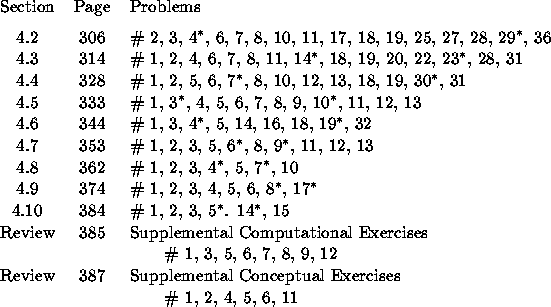Homework Problems for Chapter 4
Vector Spaces and Linear Transformations• This is the final chapter that we will be discussing. Successful mastery of this material depends critically on the understanding of the topics introduced in Chapter 2: subspace, independence, basis, dimension, ....
• The terminology is critical! If you don't understand what the question is asking, there is virtually now way to know what the question asks you to do.
• Problems marked with an asterisk () will be collected and graded. In general, problems will be due the day following the lecture in which the section was discussed.
• These problems have been selected as the minimum amount of work that you should be doing for this course. Some problems will be straightforward computations; some will require the construction of a logical argument using the terminology and concepts discussed in class; some problems will require you to expand your knowledge (with given information) and apply this to a new setting.
• If you need more practice, there are many other problems in the text that are similar to the ones I have assigned.
• There are two sections of review problems at the end of each chapter. None of these problems will be collected for grading. These problems should be a good test of your understanding of the main points in the chapter. I suggest solving these problems as one part of your preparation for an exam.
• Be sure you know how to do all of these problems. Do not hesitate to ask questions - in class, by e-mail, or during office hours.